# 原神 - 元素效果講解及前期隊伍配置推薦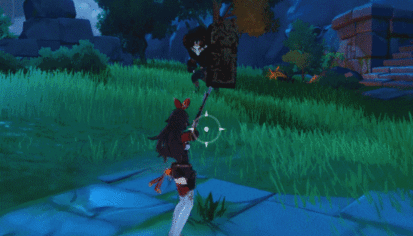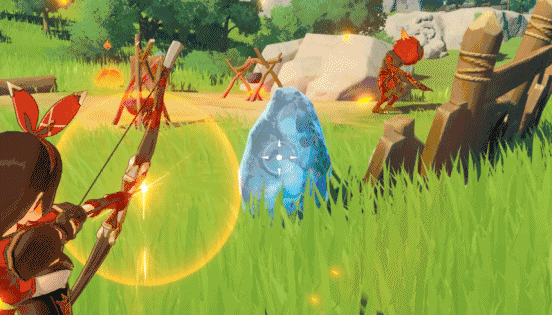(擊碎水琥珀產生雨雲。)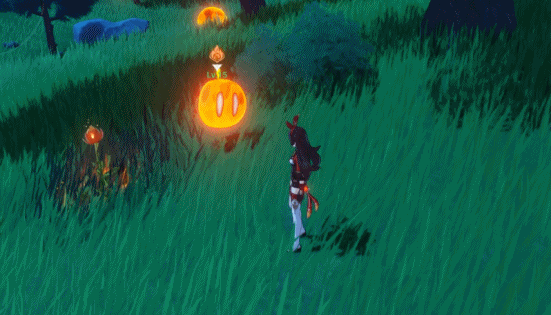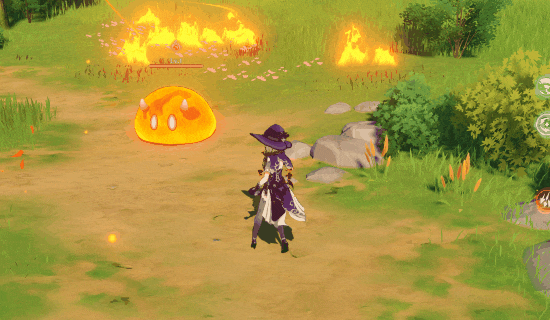• 水+冰=凍結

• 火+冰=融化

• 火+水=蒸發

• 凍結+(雙手劍)=碎冰
• 融化+雷=超載
• 蒸發+冰=凍結

• 火+草=燃燒

• 風+火/水/冰/雷=擴散

• 雷+冰=超導

• 雷+火=超載

• 雷+水=感電

• 岩+火/水/冰/雷=結晶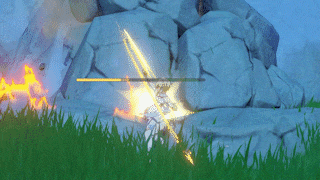(直接攻擊碎石也是可以的)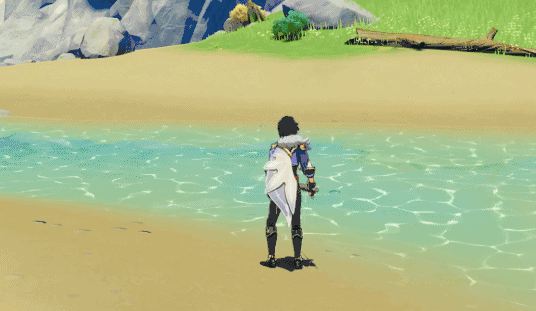(有的火把要細心才能找全)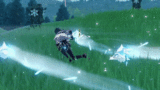風 火 水 冰 雷 岩 砂糖 迪盧克 莫娜 凱亞 麗莎 諾艾爾 琴 班尼特 芭芭拉 七七 菲謝爾 凝光 溫蒂 可莉 行秋 重雲 雷澤 魈 安柏 刻晴 香菱 北斗